saojipo.com

## 易语言画板绘制自适应表格源码

 子程序名 返回值类型 公开 备 注 create_Tabble 字节集 参数名 类 型 参考 可空 数组 备 注 区服名称 文本型 出售价格 文本型 √ 回收价格 文本型 √
 变量名 类 型 静态 数组 备 注 i 整数型 第一列数据 文本型 8 x偏移 整数型 画板1 内存画板

' 竖线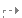计次循环首 (4, i)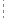画板1.画直线 ( (i － 1) × 84, 38, (i － 1) × 84, 291)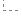计次循环尾 ()
' 横线计次循环首 (9, i)画板1.画直线 (0, i × 28 ＋ 38, 253, i × 28 ＋ 38)计次循环尾 ()
' 表头

' 第一列
i ＝ 0计次循环首 (8, i)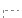判断 (i ＝ 1)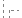x偏移 ＝ 29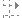判断 (i ＝ 2)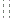x偏移 ＝ 31判断 (i ＞ 2)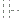x偏移 ＝ 27画板1.置写出位置 (x偏移, 43 ＋ i × 28)画板1.写文本行 (第一列数据 [i])计次循环尾 ()
i ＝ 0
' 第二列计次循环首 (8, i)判断 (i ＝ 1 i ≥ 7)x偏移 ＝ 29 ＋ 79判断 (i ≥ 2 i ＜ 4)x偏移 ＝ 31 ＋ 86判断 (i ≥ 4 i ＜ 7)x偏移 ＝ 27 ＋ 86画板1.置写出位置 (x偏移, 43 ＋ i × 28)画板1.写文本行 (出售价格 [i])计次循环尾 ()
i ＝ 0
' 第三列计次循环首 (8, i)判断 (i ＝ 1 i ≥ 7)x偏移 ＝ 29 ＋ 79 ＋ 83判断 (i ≥ 2 i ＜ 4)x偏移 ＝ 31 ＋ 86 ＋ 84判断 (i ≥ 4 i ＜ 7)x偏移 ＝ 27 ＋ 86 ＋ 82画板1.置写出位置 (x偏移, 43 ＋ i × 28)画板1.写文本行 (回收价格 [i])计次循环尾 ()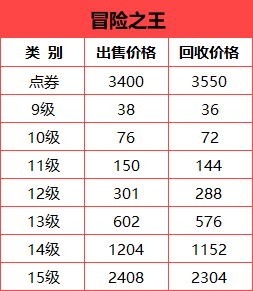## 帮网友写的一个易语言冒泡算法

 窗口程序集名 保 留 保 留 备 注 窗口程序集_启动窗口 变量名 类 型 数组 备 注 student 成绩信息 0

 子程序名 返回值类型 公开 备 注 __启动窗口_创建完毕

 子程序名 返回值类型 公开 备 注 初始化
 变量名 类 型 静态 数组 备 注 i 整数型 name 文本型 0 age 整数型 0 score 小数型 0 j 整数型
name ＝ { “张一山”, “张二川”, “张三丰”, “张四季”, “张五岳”, “张六顺”, “张七夕”, “张八戒”, “张九玲”, “张十美” }
age ＝ { 15, 17, 16, 14, 18, 16, 19, 18, 17, 16 }
score ＝ { 80, 60, 76, 89.5, 90, 90, 50, 80, 99, 78.5 }计次循环首 (取数组成员数 (name), j)
student [j].name ＝ name [j]
student [j].age ＝ age [j]
student [j].score ＝ score [j]计次循环尾 ()计次循环首 (取数组成员数 (student), i)如果 (寻找文本 (到文本 (student [i].score), “.”, , ) ＝ -1) ' 如果成绩存在小数点，那么调整加入文本格式让文本对齐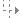编辑框1.加入文本 (“| ” ＋ student [i].name ＋ “ | ”到文本 (student [i].age)“ | ”到文本 (student [i].score)“ | ”#换行符“ —————————-”#换行符 )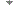计次循环尾 ()
 子程序名 返回值类型 公开 备 注 _按钮2_被单击

 子程序名 返回值类型 公开 备 注 冒泡排序
 变量名 类 型 静态 数组 备 注 i 整数型 j 整数型 age 整数型 name 文本型 score 小数型计次循环首 (取数组成员数 (student), i)变量循环首 (取数组成员数 (student) － 1, i, -1, j)如果真 (按钮2.标题 ＝ “按年龄排序” student [j ＋ 1].age ＜ student [j].age 按钮2.标题 ＝ “按成绩排序” student [j ＋ 1].score ＜ student [j].score) ' 如果前一个数比后一个数大就交换两个数组成员的值,自定义数据内其他数组索引也跟随改变
age ＝ student [j].age
name ＝ student [j].name
score ＝ student [j].score
student [j].age ＝ student [j ＋ 1].age
student [j].name ＝ student [j ＋ 1].name
student [j].score ＝ student [j ＋ 1].score
student [j ＋ 1].age ＝ age
student [j ＋ 1].name ＝ name
student [j ＋ 1].score ＝ score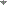变量循环尾 ()如果 (寻找文本 (到文本 (student [i].score), “.”, , ) ＝ -1)编辑框1.加入文本 (“| ” ＋ student [i].name ＋ “ | ”到文本 (student [i].age)“ | ”到文本 (student [i].score)“ | ”#换行符“ —————————-”#换行符 )计次循环尾 ()如果 (按钮2.标题 ＝ “按成绩排序”)按钮2.标题 ＝ “按成绩排序”数据类型名 公开 备 注 成绩信息 成员名 类 型 传址 数组 备 注 name 文本型 age 整数型 score 小数型

• 回顶